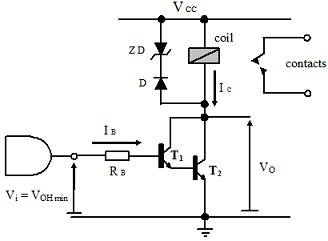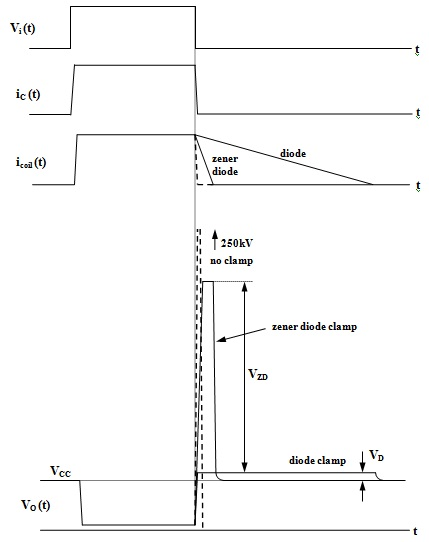#### Theory of Transistor Inverter Applications II

A Relay Driving Circuit:

A driving circuit is needed to operate the relay employed in a telephone switching exchange. The coil of relay has an inductance of 100mH and an activation current of 250mA is needed whenever operating from a 12V supply. The driving circuit is to be controlled from the output of CMOS logic gate that can source a maximum current of 1mA with a minimum output high logic voltage VOH min = 0.8VDD and that operates from a 5V supply. The deactivation time of coil (that is, time for magnetic field to liberate the contacts) must not exceed 1ms. Transistors are accessible that have a minimum βF = 50 and base-collector reverse breakdown voltage of 80V.Figure: Schematic Diagram of Relay Driver

Step 1: Find out the collector current needed in the transistor that is simply the activation current for the relay coil and hence:

IC = 250 mA

Step 2: Find out the corresponding base current needed to bring transistor to the edge of saturation whenever turned ON by the gate input as:

IBEOS = ICF = 250 mA/50 = 5 mA

When a safety overdrive factor is permitted, this will be even higher and is fine above the maximum current which the CMOS gate can offer. The current needed from the gate output should be decreased somehow.

Step 3: Solution can be found in the form of Darlington transistor configuration. In this configuration, the beta factors of two transistors are joined by employing the emitter current of one transistor as the input base current of second transistor as shown in figure above. The effective beta factor is then the product of beta factor of the two individual transistors. Minimum input base current needed to the first transistor can then be found as:

IBEOS = (IC combF comb) = (IC1 + IC2)/(βF1 βF2) = IC2F = 250 mA/2500 = 0.1 mA = 100 μA

When a reasonable overdrive factor of 3 is permitted to cater for variations in beta factors and temperature, the real base current is as follows:

IB = σL IBEOS = 3 x 100 μA = 300 μA

Step 4: The base resistor can then be computed as before supposing a minimum input voltage from the logic gate, ViH min and the fact that there are now two transistors and hence two VBE drops should be accounted for. Then,

RB = VRB/IB = (VOH min – 2 VBE sat)/IB = (0.8 x 5V – 2 x 0.8V)/300 μA = 2.4V/0.3 mA = 8 KΩ

Step 5: The problem occurs when turning the transistors off to deactivate relay. As the coil is inductive, a back emf will be developed across it whenever the current via it is altered. Whenever the current in the coil is forced to zero (0) by turning off the transistors, a back-emf will be produced across the coil that tends to oppose this modification. This back-emf will thus be in opposite direction to the voltage drop that prevailed across the coil whenever the relay was activated. The magnitude of back-emf depends on the rate at which the current is modified and can be quite big whenever the current is changed very fast. When a finite turn-off time for transistors is taken as around 100ns, the value of back-emf can be estimated as:

VLB = L (di/dt) = 100 x 10-3 x [(-250 x 10-3)/(100 x 10-9)] = -250 x 103 = -250 KV

When the 12V supply is supposed ideal, then this voltage remains fixed, that means that whenever the transistors are turned off the potential at lower end of the coil increases towards +250kV relative to ground potential. In actuality it will not go as high as this however will be several hundred volts. This will bring potential at collectors of transistors well above the reverse breakdown voltage.

The general solution to back emf problem is to join a diode in reverse across coil. This limits the back emf to –VD ON = -0.5V and thus protects the transistors. The problem with this is that, the rate at which energy can now be eliminated from the coil is limited as:

di/dt = VLB/L = - 0.5/(100 x 10-3) = -5 As-1

This signifies that to bring a current of 250mA to zero if it is supposed to decay linearly needs a time of:

T turn off = (- 250 mA)/ (- 5 As-1) = 0.25/5 = 0.05 s = 50 ms

This is much longer than the limit provided in the specification that is 1ms. The deactivation time can be decreased by permitting a higher back emf to develop across the coil whenever deactivating. As the transistors encompass a collector-base reverse breakdown voltage of 80V, a back emf approaching this could be permitted.Figure: Waveforms exhibiting Operation of the Relay Driver Circuit

This can be attained by joining a Zener diode in series with the standard diode positioned across the relay coil. To get reasonable lifetime from transistors, a Zener voltage of say 50V is reasonable. Then the revised rate of change of current can be as:

di/dt = VLB/L = -50.5/(100 x 10-3) ≈ - (50/0.1) = - 500 As-1

This provides a revised turn off time of:

T turn off = (- 250 mA/- 500 As-1) = 0.25/500 = 0.0005 s = 0.5 ms

Step 6: By an active current level as high as 0.25A, it is intelligent to check any relevant power ratings. Supposing that the given relay has been selected accurately by the user, the only thing of concern is the power dissipation in transistors. Prior to this is done, the method of operation of transistors must be looked at a little more closely.

In Darlington configuration employed, T1 is driven by the logic gate and giving it has adequate base current it will be driven into saturation and hence VCE1 = VCE sat. Base of transistor T2, on other hand, is fed from the emitter of T1. In first instance it might be supposed that provided this current was high sufficient, T2 must as well be driven into saturation. Though, it can be seen from the circuit, that with collectors of the two transistors joined together, the base-collector junction of transistor T2 can’t become forward biased. However, transistor T1, itself in saturation with VCE1 = VCE sat, maintains a least reverse bias of this amount across base-collector junction of T2 , and hence the latter remains in forward active mode with:

VCE2 = VCE1 + VBE2 = VCE sat + VBE on = 0.2V + 0.7V = 0.9V

Mainly the relay coil current flows in the collector of T2 with only a fraction going to T1. In this case, the power dissipated in T2 can be computed as:

PT2 = VCE2 x IC = 0.9V x 250 mA = 225 mW

This is too high to employ a transistor with a 250mW power rating and one with 500mW rating will outcome much a longer life-time.

Latest technology based Electrical Engineering Online Tutoring Assistance

Tutors, at the www.tutorsglobe.com, take pledge to provide full satisfaction and assurance in Electrical Engineering help via online tutoring. Students are getting 100% satisfaction by online tutors across the globe. Here you can get homework help for Electrical Engineering, project ideas and tutorials. We provide email based Electrical Engineering help. You can join us to ask queries 24x7 with live, experienced and qualified online tutors specialized in Electrical Engineering. Through Online Tutoring, you would be able to complete your homework or assignments at your home. Tutors at the TutorsGlobe are committed to provide the best quality online tutoring assistance for Electrical Engineering Homework help and assignment help services. They use their experience, as they have solved thousands of the Electrical Engineering assignments, which may help you to solve your complex issues of Electrical Engineering. TutorsGlobe assure for the best quality compliance to your homework. Compromise with quality is not in our dictionary. If we feel that we are not able to provide the homework help as per the deadline or given instruction by the student, we refund the money of the student without any delay.

2015 ©TutorsGlobe All rights reserved. TutorsGlobe Rated 4.8/5 based on 34139 reviews.